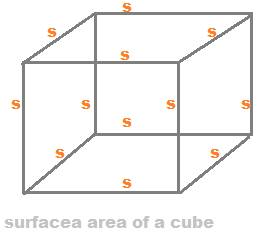## Surface area of a cubeSurface area of a cube is of two types. One is the

1. Total surface area of cube and the other is
2. Lateral surface area of the cube.

A cube is a solid shape or a 3d figure, in which all the six sides are equal in length.

The sides are also called as edges or dimensions.

Since all the 12 sides are equal in length, therefore every face is a square. Thus, a cube is formed of six square shaped faces joined end to end.

The Lateral Surface Area of a Cube:

This surface area of a cube includes four square faces:

The two side faces and the front and back faces.

So, lateral surface area of a cube is 4s2.

The Total Surface Area of a Cube:

This surface area includes all the six square faces of a cube.

Since every face is square shaped of a same area s2, so total surface area of a cube is 12s2

Example 1:

Find both the lateral surface area of a cube and the total surface of cube in which the diagonal length is 4√3.

Solution:

In a cube, the length of a diagonal is s×√3, where s is length of the side of the cube.
So, s×√3 = 4×√3, hence s = 4.

Therefore,

Lateral surface area of cube = 4s2 = 4 × 42 = 64 sq. units, and

Total surface area of cube = 6s2 = 6× 42 = 96 sq. units.